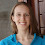### Predictions of ACE's surveying results

Carl Shulman is polling people about their predictions for the results of the upcoming ACE study to encourage less biased interpretations. Here are mine.

Assuming control group follows the data in e.g. the Iowa Women's Health Study they should eat 166g meat/day with sd 66g.1 (For the rest of this post, I'm going to assume everything is normally distributed, even though I realize that's not completely true.)

For mathematical ease, let's take our prior from the farm sanctuary study and say: 2% are now veg, and an additional 5% eat "a lot less" meat which I'll define as cutting in half. So the mean of this group is 159g (4.2% less) w/ sd 69g.

I don't know what tests they will do, but let's look at a t-test because that's easiest. The test statistic here is:
$$t=\frac{166-159}{\sqrt{\frac{66}{N_1}+\frac{69}{N_2}}}$$
Let's assume 5% of those surveyed were in the intervention group. Solving for $N$ in
$$1.96=\frac{7}{\sqrt{\frac{66}{.95N}+\frac{69}{.05N}}}$$
we find $N\approx 350$, meaning that I expect the null hypothesis to be rejected at the usual $\alpha=.05$ if they collected at least 350 survey responses.2 I'm leaning slightly towards it not being significant, but I'm not sure how much data they collected.

Here's my estimate of their estimate (I can't do this analytically, so this is based on simulations):
You can see that the expected outcome is the true difference of about 4 veg equivalents per 100 leaflets, but with such a small sample size there is a 25% chance that we'll find leafleted people were less likely to go veg.

Here's how a 50% confidence interval might shake out:

The left graph is the bottom of the CI, the right one is the top.

### Putting Money where my Mouth Is

The point of this is so that I don't retro-justify my beliefs, which is that meta-research in animal-related fields is the most effective thing. I have a lot of model uncertainty, but I would broadly endorse the conclusions of the above. The following represent ~2.5% probability events (each), which I will take as evidence I'm wrong.
• If a 50% CI is exclusively above 9 veg equivalents per 100 leaflets, then I think its ability to attract people to veganism outweighs the knowledge we'd gain from more studies. Therefore, I pledge $1,000 to VO or THL (or whatever top-ranked leafleting charity exists at the time). • If a 50% CI is exclusively below zero, then veg interventions in general are less useful than I thought. Therefore I pledge$1,000 to MIRI (or another x-risk charity, if e.g. GiveWell Labs has a recommendation by then).
I don't think my above model is completely correct, and I'm sure ACE will have a different parameterization, so I don't know that these are really the 5% tails, but I would consider either of them to be a surprising enough event that my current beliefs are probably wrong.

I am open to friendly charity bets (if result is worse than X I give money to your charity, else you give to mine), if anyone else is interested.

Footnotes
1. I tried to use MLE to combine multiple analyses, but found that the standard deviation is > 10,000 g/day. It's a good thing ACE has professional statisticians on the job, because the data clearly is kind of complex.
2. I used $d.f.=\infty$

1.Given that the study was longitudinal, do you have predictions for the rate of change in diet for the control group and treatment group?

Where would you donate in the 95% case that doesn't involve the two tails?

1.By "rate of change", do you mean predictions about how quickly someone switched their diet? Even after the results are in, I don't think we'll be able to tell that.

I suggested he donate to EAA in the case that it confirms his belief that meta-research for animal charities is most effective.

2.Where did you get that 5% of people eat "a lot less" meat after receiving a leaflet? The farm sanctuary study says 7-12% claimed to eat a lot less meat.